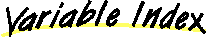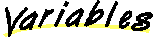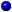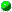`All Packages  Class Hierarchy  This Package  Previous  Next  Index`

# Class java.math.BigDecimal

```java.lang.Object
|
+----java.lang.Number
|
+----java.math.BigDecimal
```

public class BigDecimal
extends Number
Immutable, arbitrary-precision signed decimal numbers. A BigDecimal consists of an arbitrary precision integer value and a non-negative integer scale, which represents the number of decimal digits to the right of the decimal point. (The number represented by the BigDecimal is intVal/10**scale.) BigDecimals provide operations for basic arithmetic, scale manipulation, comparison, format conversion and hashing.

The BigDecimal class gives its user complete control over rounding behavior, forcing the user to explicitly specify a rounding behavior for operations capable of discarding precision (divide and setScale). Eight rounding modes are provided for this purpose. Two types of operations are provided for manipulating the scale of a BigDecimal: scaling/rounding operations and decimal point motion operations. Scaling/Rounding operations (SetScale) return a BigDecimal whose value is approximately (or exactly) equal to that of the operand, but whose scale is the specified value; that is, they increase or decrease the precision of the number with minimal effect on its value. Decimal point motion operations (movePointLeft and movePointRight) return a BigDecimal created from the operand by moving the decimal point a specified distance in the specified direction; that is, they change a number's value without affecting its precision.

BigInteger

##ROUND_CEILING
If the BigDecimal is positive, behave as for ROUND_UP; if negative, behave as for ROUND_DOWN.ROUND_DOWN
Never increment the digit prior to a discarded fraction (i.e., truncate).ROUND_FLOOR
If the BigDecimal is positive, behave as for ROUND_DOWN; if negative behave as for ROUND_UP.ROUND_HALF_DOWN
Behave as for ROUND_UP if the discarded fraction is > .5; otherwise, behave as for ROUND_DOWN.ROUND_HALF_EVEN
Behave as for ROUND_HALF_UP if the digit to the left of the discarded fraction is odd; behave as for ROUND_HALF_DOWN if it's even.ROUND_HALF_UP
Behave as for ROUND_UP if the discarded fraction is >= .5; otherwise, behave as for ROUND_DOWN.ROUND_UNNECESSARY
This "pseudo-rounding-mode" is actually an assertion that the requested operation has an exact result, hence no rounding is necessary.ROUND_UP
Always increment the digit prior to a non-zero discarded fraction.

##BigDecimal(BigInteger)
Translates a BigInteger into a BigDecimal.BigDecimal(BigInteger, int)
Translates a BigInteger and a scale into a BigDecimal.BigDecimal(double)
Translates a double into a BigDecimal.BigDecimal(String)
Constructs a BigDecimal from a string containing an optional minus sign followed by a sequence of zero or more decimal digits, optionally followed by a fraction, which consists of a decimal point followed by zero or more decimal digits.

##abs()
Returns a BigDecimal whose value is the absolute value of this number, and whose scale is this.scale().add(BigDecimal)
Returns a BigDecimal whose value is (this + val), and whose scale is MAX(this.scale(), val.scale).compareTo(BigDecimal)
Returns -1, 0 or 1 as this number is less than, equal to, or greater than val.divide(BigDecimal, int)
Returns a BigDecimal whose value is (this / val), and whose scale is this.scale().divide(BigDecimal, int, int)
Returns a BigDecimal whose value is (this / val), and whose scale is as specified.doubleValue()
Converts the number to a double.equals(Object)
Returns true iff x is a BigDecimal whose value is equal to this number.floatValue()
Converts this number to a float.hashCode()
Computes a hash code for this object.intValue()
Converts this number to an int.longValue()
Converts this number to a long.max(BigDecimal)
Returns the BigDecimal whose value is the greater of this and val.min(BigDecimal)
Returns the BigDecimal whose value is the lesser of this and val.movePointLeft(int)
Returns a BigDecimal which is equivalent to this one with the decimal point moved n places to the left.movePointRight(int)
Moves the decimal point the specified number of places to the right.multiply(BigDecimal)
Returns a BigDecimal whose value is (this * val), and whose scale is this.scale() + val.scale.negate()
Returns a BigDecimal whose value is -1 * this, and whose scale is this.scale().scale()
Returns the scale of this number.setScale(int)
Returns a BigDecimal whose scale is the specified value, and whose value is exactly equal to this number's.setScale(int, int)
Returns a BigDecimal whose scale is the specified value, and whose integer value is determined by multiplying or dividing this BigDecimal's integer value by the appropriate power of ten to maintain the overall value.signum()
Returns the signum function of this number (i.e., -1, 0 or 1 as the value of this number is negative, zero or positive).subtract(BigDecimal)
Returns a BigDecimal whose value is (this - val), and whose scale is MAX(this.scale(), val.scale).toBigInteger()
Converts this number to a BigInteger.toString()
Returns the string representation of this number.valueOf(long)
Returns a BigDecimal with the given value and a scale of zero.valueOf(long, int)
Returns a BigDecimal with a value of (val/10**scale).

##ROUND_UP
``` public static final int ROUND_UP
```
Always increment the digit prior to a non-zero discarded fraction. Note that this rounding mode never decreases the magnitude. (Rounds away from zero.)ROUND_DOWN
``` public static final int ROUND_DOWN
```
Never increment the digit prior to a discarded fraction (i.e., truncate). Note that this rounding mode never increases the magnitude. (Rounds towards zero.)ROUND_CEILING
``` public static final int ROUND_CEILING
```
If the BigDecimal is positive, behave as for ROUND_UP; if negative, behave as for ROUND_DOWN. Note that this rounding mode never decreases the value. (Rounds towards positive infinity.)ROUND_FLOOR
``` public static final int ROUND_FLOOR
```
If the BigDecimal is positive, behave as for ROUND_DOWN; if negative behave as for ROUND_UP. Note that this rounding mode never increases the value. (Rounds towards negative infinity.)ROUND_HALF_UP
``` public static final int ROUND_HALF_UP
```
Behave as for ROUND_UP if the discarded fraction is >= .5; otherwise, behave as for ROUND_DOWN. (Rounds towards "nearest neighbor" unless both neighbors are equidistant, in which case rounds up.)ROUND_HALF_DOWN
``` public static final int ROUND_HALF_DOWN
```
Behave as for ROUND_UP if the discarded fraction is > .5; otherwise, behave as for ROUND_DOWN. (Rounds towards "nearest neighbor" unless both neighbors are equidistant, in which case rounds down.)ROUND_HALF_EVEN
``` public static final int ROUND_HALF_EVEN
```
Behave as for ROUND_HALF_UP if the digit to the left of the discarded fraction is odd; behave as for ROUND_HALF_DOWN if it's even. (Rounds towards the "nearest neighbor" unless both neighbors are equidistant, in which case, rounds towards the even neighbor.)ROUND_UNNECESSARY
``` public static final int ROUND_UNNECESSARY
```
This "pseudo-rounding-mode" is actually an assertion that the requested operation has an exact result, hence no rounding is necessary. If this rounding mode is specified on an operation that yields an inexact result, an arithmetic exception is thrown.

##BigDecimal
``` public BigDecimal(String val) throws NumberFormatException
```
Constructs a BigDecimal from a string containing an optional minus sign followed by a sequence of zero or more decimal digits, optionally followed by a fraction, which consists of a decimal point followed by zero or more decimal digits. The string must contain at least one digit in the integer or fractional part. The scale of the resulting BigDecimal will be the number of digits to the right of the decimal point in the string, or 0 if the string contains no decimal point. The character-to-digit mapping is provided by Character.digit. Any extraneous characters (including whitespace) will result in a NumberFormatException.BigDecimal
``` public BigDecimal(double val) throws NumberFormatException
```
Translates a double into a BigDecimal. The scale of the BigDecimal is the smallest value such that (10**scale * val) is an integer. A double whose value is -infinity, +infinity or NaN will result in a NumberFormatException.BigDecimal
``` public BigDecimal(BigInteger val)
```
Translates a BigInteger into a BigDecimal. The scale of the BigDecimal is zero.BigDecimal
``` public BigDecimal(BigInteger val,
int scale) throws NumberFormatException
```
Translates a BigInteger and a scale into a BigDecimal. The value of the BigDecimal is (BigInteger/10**scale). A negative scale will result in a NumberFormatException.

##valueOf
``` public static BigDecimal valueOf(long val,
int scale) throws NumberFormatException
```
Returns a BigDecimal with a value of (val/10**scale). This factory is provided in preference to a (long) constructor because it allows for reuse of frequently used BigDecimals (like 0 and 1), obviating the need for exported constants. A negative scale will result in a NumberFormatException.valueOf
``` public static BigDecimal valueOf(long val)
```
Returns a BigDecimal with the given value and a scale of zero. This factory is provided in preference to a (long) constructor because it allows for reuse of frequently used BigDecimals (like 0 and 1), obviating the need for exported constants.add
``` public BigDecimal add(BigDecimal val)
```
Returns a BigDecimal whose value is (this + val), and whose scale is MAX(this.scale(), val.scale).subtract
``` public BigDecimal subtract(BigDecimal val)
```
Returns a BigDecimal whose value is (this - val), and whose scale is MAX(this.scale(), val.scale).multiply
``` public BigDecimal multiply(BigDecimal val)
```
Returns a BigDecimal whose value is (this * val), and whose scale is this.scale() + val.scale.divide
``` public BigDecimal divide(BigDecimal val,
int scale,
int roundingMode) throws ArithmeticException, IllegalArgumentException
```
Returns a BigDecimal whose value is (this / val), and whose scale is as specified. If rounding must be performed to generate a result with the given scale, the specified rounding mode is applied. Throws an ArithmeticException if val == 0, scale < 0, or the rounding mode is ROUND_UNNECESSARY and the specified scale is insufficient to represent the result of the division exactly. Throws an IllegalArgumentException if roundingMode does not represent a valid rounding mode.divide
``` public BigDecimal divide(BigDecimal val,
int roundingMode) throws ArithmeticException, IllegalArgumentException
```
Returns a BigDecimal whose value is (this / val), and whose scale is this.scale(). If rounding must be performed to generate a result with the given scale, the specified rounding mode is applied. Throws an ArithmeticException if val == 0. Throws an IllegalArgumentException if roundingMode does not represent a valid rounding mode.abs
``` public BigDecimal abs()
```
Returns a BigDecimal whose value is the absolute value of this number, and whose scale is this.scale().negate
``` public BigDecimal negate()
```
Returns a BigDecimal whose value is -1 * this, and whose scale is this.scale().signum
``` public int signum()
```
Returns the signum function of this number (i.e., -1, 0 or 1 as the value of this number is negative, zero or positive).scale
``` public int scale()
```
Returns the scale of this number.setScale
``` public BigDecimal setScale(int scale,
int roundingMode) throws ArithmeticException, IllegalArgumentException
```
Returns a BigDecimal whose scale is the specified value, and whose integer value is determined by multiplying or dividing this BigDecimal's integer value by the appropriate power of ten to maintain the overall value. If the scale is reduced by the operation, the integer value must be divided (rather than multiplied), and precision may be lost; in this case, the specified rounding mode is applied to the division. Throws an ArithmeticException if scale is negative, or the rounding mode is ROUND_UNNECESSARY and it is impossible to perform the specified scaling operation without loss of precision. Throws an IllegalArgumentException if roundingMode does not represent a valid rounding mode.setScale
``` public BigDecimal setScale(int scale) throws ArithmeticException, IllegalArgumentException
```
Returns a BigDecimal whose scale is the specified value, and whose value is exactly equal to this number's. Throws an ArithmeticException if this is not possible. This call is typically used to increase the scale, in which case it is guaranteed that there exists a BigDecimal of the specified scale and the correct value. The call can also be used to reduce the scale if the caller knows that the number has sufficiently many zeros at the end of its fractional part (i.e., factors of ten in its integer value) to allow for the rescaling without loss of precision. Note that this call returns the same result as the two argument version of setScale, but saves the caller the trouble of specifying a rounding mode in cases where it is irrelevant.movePointLeft
``` public BigDecimal movePointLeft(int n)
```
Returns a BigDecimal which is equivalent to this one with the decimal point moved n places to the left. If n is non-negative, the call merely adds n to the scale. If n is negative, the call is equivalent to movePointRight(-n). (The BigDecimal returned by this call has value (this * 10**-n) and scale MAX(this.scale()+n, 0).)movePointRight
``` public BigDecimal movePointRight(int n)
```
Moves the decimal point the specified number of places to the right. If this number's scale is >= n, the call merely subtracts n from the scale; otherwise, it sets the scale to zero, and multiplies the integer value by 10 ** (n - this.scale). If n is negative, the call is equivalent to movePointLeft(-n). (The BigDecimal returned by this call has value (this * 10**n) and scale MAX(this.scale()-n, 0).)compareTo
``` public int compareTo(BigDecimal val)
```
Returns -1, 0 or 1 as this number is less than, equal to, or greater than val. Two BigDecimals that are equal in value but have a different scale (e.g., 2.0, 2.00) are considered equal by this method. This method is provided in preference to individual methods for each of the six boolean comparison operators (<, ==, >, >=, !=, <=). The suggested idiom for performing these comparisons is: (x.compareTo(y) 0), where is one of the six comparison operators.equals
``` public boolean equals(Object x)
```
Returns true iff x is a BigDecimal whose value is equal to this number. This method is provided so that BigDecimals can be used as hash keys. Unlike compareTo, this method considers two BigDecimals equal only if they are equal in value and scale.

Overrides:
equals in class Objectmin
``` public BigDecimal min(BigDecimal val)
```
Returns the BigDecimal whose value is the lesser of this and val. If the values are equal (as defined by the compareTo operator), either may be returned.max
``` public BigDecimal max(BigDecimal val)
```
Returns the BigDecimal whose value is the greater of this and val. If the values are equal (as defined by the compareTo operator), either may be returned.hashCode
``` public int hashCode()
```
Computes a hash code for this object. Note that two BigDecimals that are numerically equal but differ in scale (e.g., 2.0, 2.00) will not generally have the same hash code.

Overrides:
hashCode in class ObjecttoString
``` public String toString()
```
Returns the string representation of this number. The digit-to- character mapping provided by Character.forDigit is used. The minus sign and decimal point are used to indicate sign and scale. (This representation is compatible with the (String, int) constructor.)

Overrides:
toString in class ObjecttoBigInteger
``` public BigInteger toBigInteger()
```
Converts this number to a BigInteger. Standard narrowing primitive conversion as per The Java Language Specification. In particular, note that any fractional part of this number will be truncated.intValue
``` public int intValue()
```
Converts this number to an int. Standard narrowing primitive conversion as per The Java Language Specification. In particular, note that any fractional part of this number will be truncated.

Overrides:
intValue in class NumberlongValue
``` public long longValue()
```
Converts this number to a long. Standard narrowing primitive conversion as per The Java Language Specification. In particular, note that any fractional part of this number will be truncated.

Overrides:
longValue in class NumberfloatValue
``` public float floatValue()
```
Converts this number to a float. Similar to the double-to-float narrowing primitive conversion defined in The Java Language Specification: if the number has too great a magnitude to represent as a float, it will be converted to infinity or negative infinity, as appropriate.

Overrides:
floatValue in class NumberdoubleValue
``` public double doubleValue()
```
Converts the number to a double. Similar to the double-to-float narrowing primitive conversion defined in The Java Language Specification: if the number has too great a magnitude to represent as a double, it will be converted to infinity or negative infinity, as appropriate.

Overrides:
doubleValue in class Number

`All Packages  Class Hierarchy  This Package  Previous  Next  Index`